MAT331 homework problems
17.
(expires 18 March)     Consider the differential equation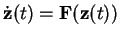, where the vector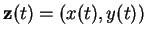and the field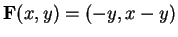. Plot a few solutions. What happens to them when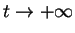? Give a Maple-proof'' that this is a general fact for every solution. [A Maple-proof'' is an argument that is rigorous once we accept Maple results as incontrovertibly true.]

18.
(expires 18 March)    (No Maple.) For the equation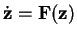,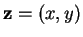, with the vector field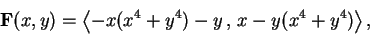prove that the origin is an attractor in the future, i.e., every solution verifies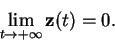[You can ask around how to do this, but then you have to show clearly that you have understood it.]

19.
(expires 18 March)     We will study the Lotke-Volterra predator-prey equations: In a very simple ecosystem, at the time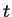(which is expressed, say, in years), there is a population of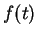foxes and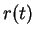rabbits. The evolution of these quantities obeys the system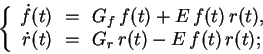where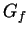and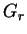are the growth rates for the foxes and the rabbits, respectively, in the absence of each other.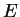is the probability of a fatal encounter between a fox and a rabbit (normalized per number of foxes and rabbits).

First, write some words to explain why these equations make sense. Then, fix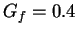,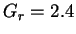(it's notorius that rabbits have the tendency to reproduce quickly) and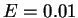. For a few initial conditions of your choice, plot the trajectories in the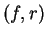-plane (say, with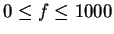and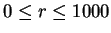). For the same initial conditions, plot the actual solutions too (i.e,against, andagainst). Write some comments interpreting how the behaviour of the solutions relates to what happens to the two species.

Finally, repeat the same procedure with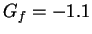. Things change substantially. Again, what is the physical'' interpretation of this?